## 20141108

### Physics presentation: wavesWatch as the very end of this whip cracks and breaks the sound barrier. As for me, when I look at this whip cracking...I'm thinking, dinosaur butt. (Video link: "WorldWideWhips introducing the Whip-Cam.")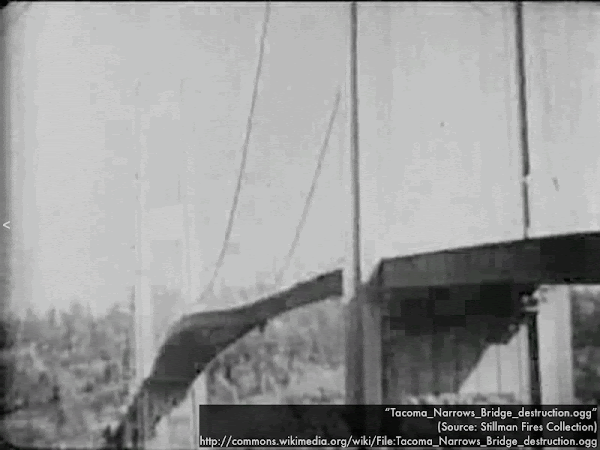This was the infamous Tacoma Narrows Bridge, where high winds set up standing waves that, unchecked, eventually destroyed it. They don't build bridges like that these days...or do they? (Video link: "Tacoma_Narrows_Bridge_destruction.ogg.")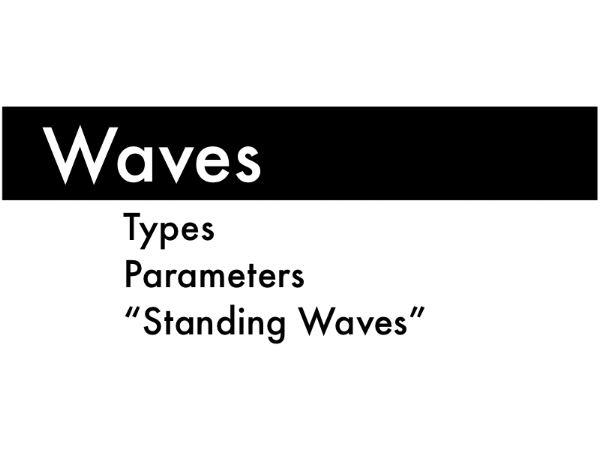We'll introduce different wave phenomena, parameters that specifically describe periodic waves, and consider "standing waves."First, and overview of different types of one-dimensional waves.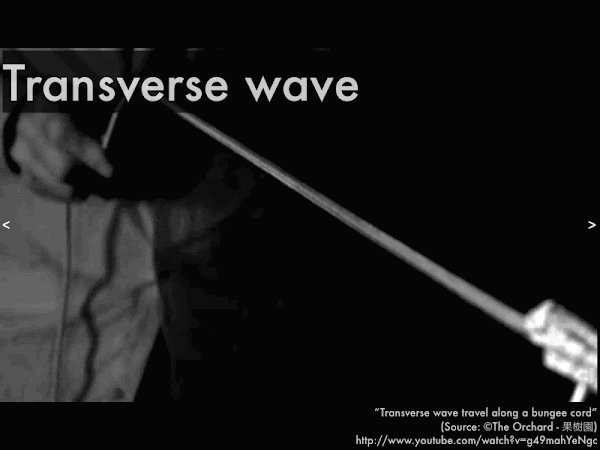For a transverse wave (here, a pulse) the disturbance is sideways to the direction of the wave motion. (Video link: "Transverse wave travel along a bungee cord.")For a longitudinal wave (here, also a pulse) the disturbance is along the direction of the wave motion. (Video link: "Longitudinal waves in a spring in slow motion.")If the disturbance (here, transverse) repeats itself periodically, then a periodic wave is set up along this rope. (Video link: "Transverse Waves.")If the disturbance (here, longitudinal) repeats itself periodically, then a periodic wave is set up along this spring. (Video link: "Transverse & Longitudinal Waves.")Next, we'll focus on waves on strings (and ropes, cables, and other similar media), and specifically on periodic waves along strings.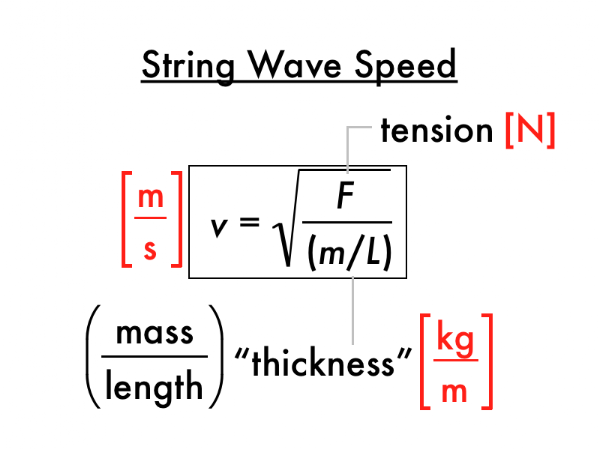The speed of a transverse pulse or transverse periodic wave along a strong depends on the square root of the string tension (here denoted by F rather than T, which we'll reserve for period, as well as for temperature near the end of this course) divided by the linear mass density, essentially the "thickness" (mass per unit length) of the string.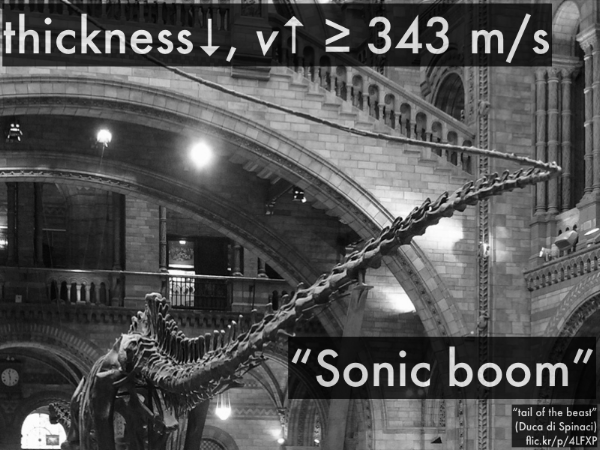Which leads us to whips and dinosaur tails. A whip must be constructed that it tapers with decreasing thickness, such that as its mass per unit length (m/L) decreases, then the wave speed increases (assuming that tension remains approximately constant). If the wave speed at the end of the whip is faster than the speed of sound (nominally 343 m/s in air), then that part of the whip will move fast enough to break the sound barrier and create a sonic boom. Computer models of certain dinosaurs indicate that their tails may have been used as whips for defense and/or signaling.The speed of periodic waves along strings is set by the string tension and thickness, but the frequency of the wave is set by the source. The resulting spatial repeat interval is the wavelength.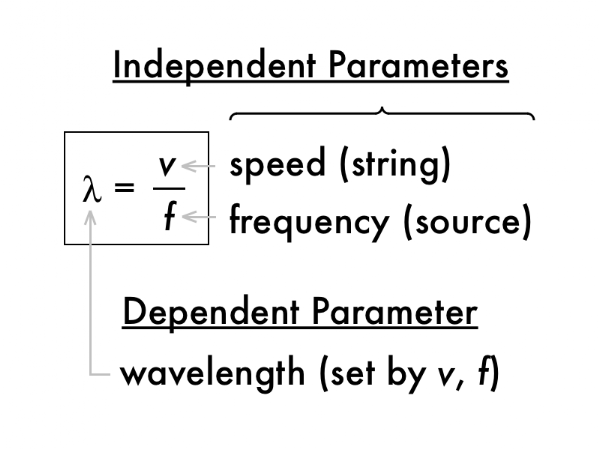Note the hierarchy of these wave parameters. Since the wave speed is determined by properties of the string (independent of the source), and the frequency is determined by the source (independent of the string), these are said to be independent wave parameters. In contrast, the wavelength of the wave is dependent on both the independent speed and frequency parameters. Algebraically there is nothing wrong with expressing this relation as v = λf and f = v/λ, as long as you recognize that the dependency of λ doesn't change.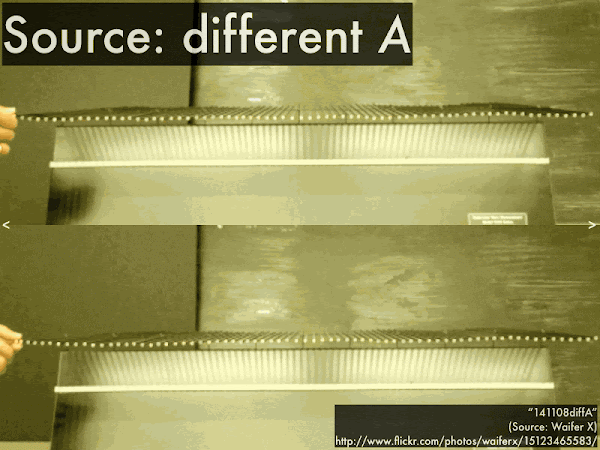The hand on the left oscillates up-and-down as the source of a wave that travels left-to-right along this apparatus. The top case is where the hand oscillates up-and-down with a certain frequency and a small amplitude, while the bottom case is where the hand oscillates up-and-down with the same frequency and larger amplitude. Which wave travels with the faster speed? Which wave has the longer wavelength? (Video link: "141108diffA.")

Let's take a look at the frequency, which was stated to be the same for both these waves, but check if that really is the case. You'll need a friend to watch this animation with you. For the top wave, every time you see the hand at the left moves up to make a crest (or "hump"), say "now." "Now. Now. Now..." Keep doing that. For the bottom wave, convince your friend to say "now" every time the hand at the left moves up to make a crest there as well." "Now. Now. Now..." If the two of you do this correctly, the rate that you say "now" should more or less be the same rate that your friend says "now." This means that these waves should have (approximately) the same frequency.

To see that the speed is the same for both these waves let's time how long each wave takes to travel across the screen from left-to-right. Watch when a crest (or "hump") starts at the left for both waves, and then say "go." You'll watch the top wave crest move all the way across to the right end of the apparatus, while your friend will watch the bottom wave crest move all the way across to the left end of the apparatus. When your wave crest reaches the right end of the apparatus, stay "stop." There should be a tie (more or less) for the time it takes for these wave crests to travel from left to right, and since they travel the same distance in the same amount of time, then they must have (approximately) the same speed.

For the wavelength, note the horizontal distance from crest-to-crest for the top wave, and mark it with two of your fingers. Compare it to the horizontal distance from crest-to-crest for the bottom wave, which your friend can also mark with two fingers. This horizontal crest-to-crest distance should be more or less the same. This means that these waves have (approximately) the same wavelength.

In this experiment, amplitude of the two waves was different, while the frequency of the two was the same. The speed of the two waves was not affected by the difference in amplitude; and the wavelength of the two waves was also not affected by the difference in amplitude. Thus both wave speed and the wavelength do not depend on changes in amplitude, and wave speed and wavelength are both independent of the amplitude of a wave, whatever it is.Here the hand oscillates up-and-down with the same amplitude, but the top case has a lower frequency and the bottom case has a higher frequency. Which wave travels with the faster speed? Which wave has the longer wavelength? (Video link: "141108difff.")In this setup, the hand oscillates up-and-down with a given frequency and amplitude. As the wave travels from left-to-right, it is then transferred to another section that allows the wave to travel at a much greater speed. Along which section does the wave have a higher frequency? Along which section does the wave have a longer wavelength? (Video link: "Wavelength & Frequency: Different Media.")Third: "standing waves," which we'll discuss in terms of resonance, rather than the more involved approach of wave superposition and reflections.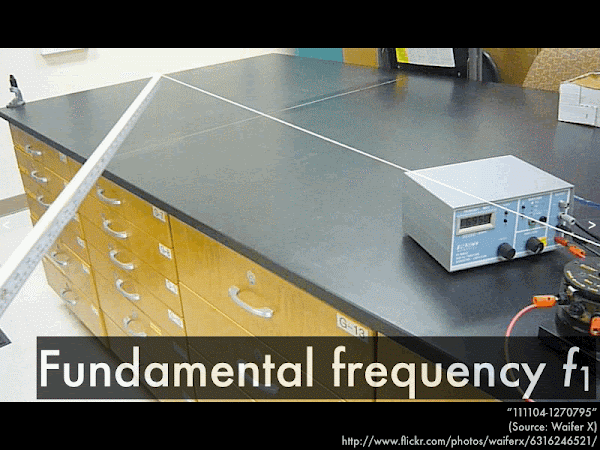A string of finite length will naturally oscillate at its fundamental frequency f1 if it is plucked. (Video link: "111104-1270795.")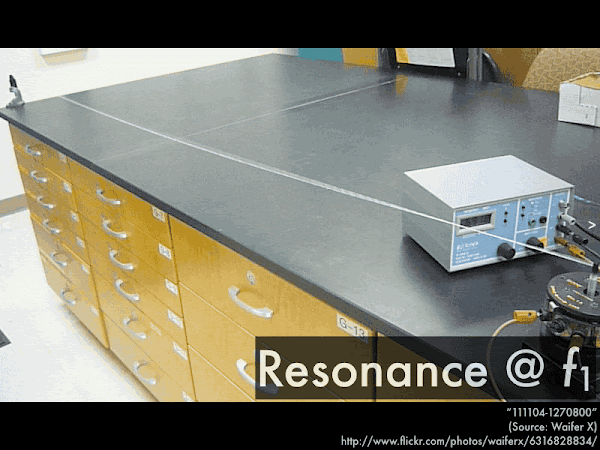If this string is periodically disturbed at the same frequency as its fundamental frequency, then the string will undergo resonance, as the timing of the periodic disturbances matches the natural vibration of the string. (Video link: "111104-1270800.")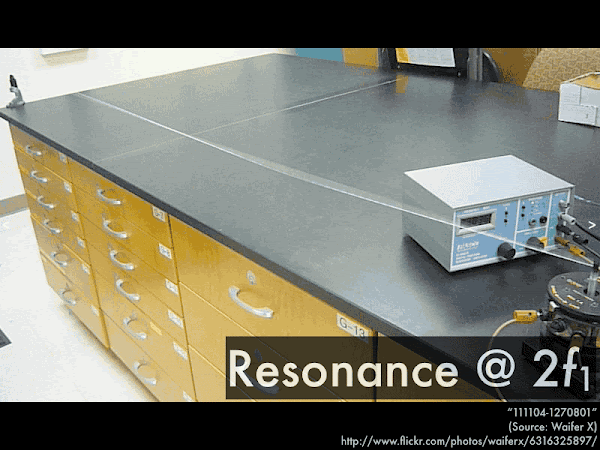You can also set the string into another resonance by oscillating it at exactly twice the fundamental frequency, this results in an interesting pattern where there is a node at the center, where the string is always fixed. This is where the "standing" in standing waves comes from. (Video link: "111104-1270801.")And same goes for oscillating the string at exactly three times the fundamental frequency (resulting in two equally spaced nodes), and so on. (Video link: "111104-1270803.")It can be shown from a variety of proofs that the fundamental frequency of a string depends on the wave speed v (which depends on its tension and thickness), and length L. The frequencies that this string will resonate at are then merely integer multiples of the fundamental frequency.In order to play different notes on a guitar, each string, while the same length, has different thicknesses (assuming that tensions are approximately equal), such that each string will vibrate at different fundamental frequencies when plucked. Would the thinnest or thickest strings have the slowest wave speed? Would the thinnest or thickest strings have the lowest fundamental frequency? (Video link: "Slow Motion GUITAR Strings -2000/4000% slower.")In order to play a larger variety of different notes, a finger will hold down a string at a certain point between its ends, effectively shortening its length, and thus changing its fundamental frequency. Would decreasing the length of a string increase or decrease its wave speed? Would decreasing the length of a string increase or decrease its fundamental frequency? (Video link: "Music in slow motion - Guitar, Bass, Drum Kit, Piano and Violin.")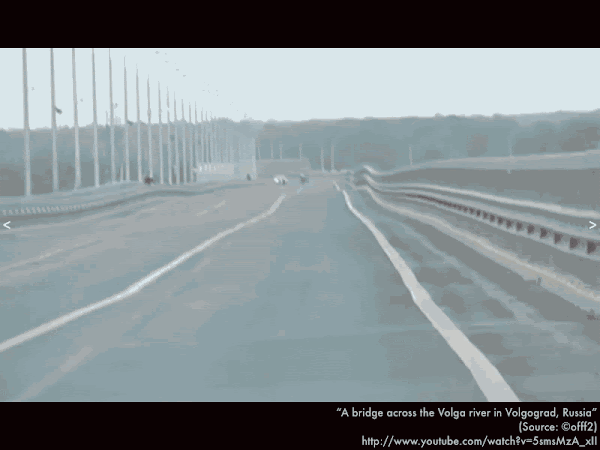Which leads us to this bridge in Volgograd, Russia. High winds buffeting this bridge have apparently excited a very high resonant frequency along it--observe the pedestrian: is he on a node or antinode? (Video link: "A bridge across the Volga river in Volgograd, Russia.")